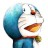# 杰拉斯的博客

## [ACM_NYOJ_2]括号匹配（一）杰拉斯 | 时间：2012-04-18, Wed | 16,559 views

### 括号配对问题

```3
[(])
(])
([[]()])
```

```No
No
Yes
```

#### 来源

NYOJ2

```#include<iostream>
#include<string>
using namespace std;

int main(){
int t;
cin >> t;
while(t--){
string s;
cin >> s;
int num = 0;
while(s.length() >= 2 && num != s.length()){
num = s.length();
for(int i = 0;i < s.length(); ++i){
if((s[i] == '(' && s[i + 1] == ')') || (s[i] == '[' && s[i + 1] == ']')){
s.erase(s.begin() + i, s.begin() + i + 2);
}
}
}
if(s.length() == 0){
cout << "Yes" << endl;
}else{
cout << "No" << endl;
}
}
return 0;
}
```